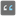• # Check solution bug?

## Question related to missionSum by Type

Hi, my solution is:

```from typing import Tuple
def sum_by_types(items: list) -> Tuple[str, int]:
return ''.join([_ for _ in items if isinstance(_, (str))]), sum([_ for _ in items if isinstance(_, (int))])
```

it passes all asserts including <sum_by_types([]) == ("", 0)>, but solution check result is: